# SSAS: Discipline, Accuracy, Attention to Details

Introduction:

Period average

It was necessary to calculate the average value for a period. In MS Analysis Services, there is the Average of Children function for this purpose, which counts the average of all non-empty values for the selected period.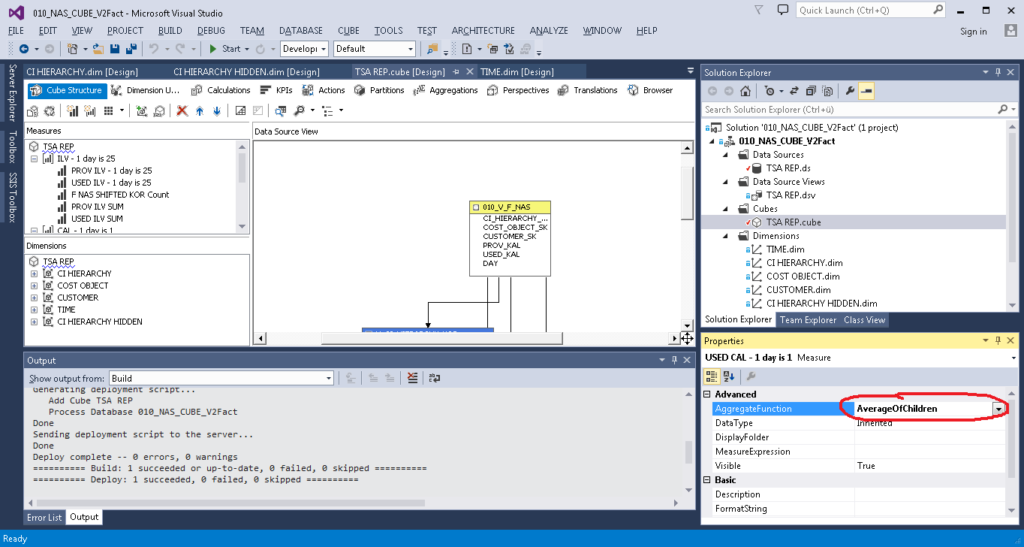But the customer, after careful examination of the results, got questions. He showed me the following sample and said that it was incorrect: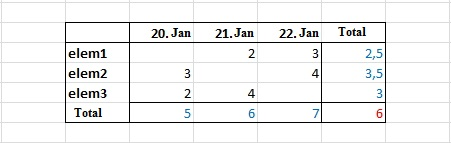Since, in his opinion, it should be like this: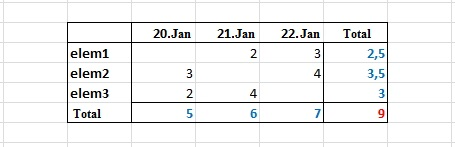To my question: Why? He replied that he needed not the average value for the period, but the sum of the average values for each element for the period, i.e:

NOT THIS WAY: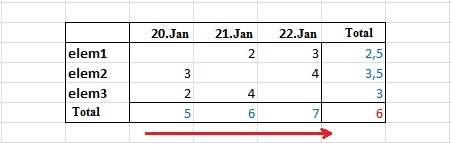(5 + 6 + 7) / 3 = 6

BUT LIKE THIS: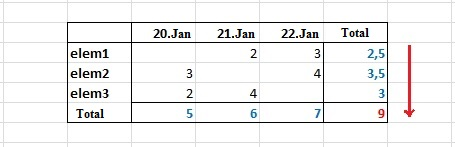(2.5 + 3.5 + 3) = 9

This desire made me go through all the stages of accepting the inevitable:

1. Denial (This is not the period average);
2. Anger (Who taught him math?);
3. Negotiation (Let’s leave as is and ask those who will use this);
4. Depression (They said that here all are very kind and good…);
5. Acceptance (If you must, you must. I will do as they asked me to do).

The decision was hardly obvious. In the Calculations section, I created Calculated Member and wrote an expression using functions iif, isleaf, and sum.

First option:

where [ELEM] is the measurement, and [ELEM SK] is its key.

The result was unexpected: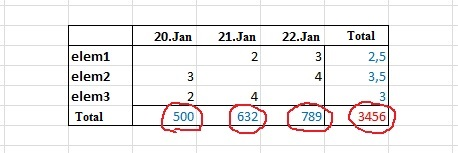The strange numbers in the Results turned out to be the sums of all the values of the elements in the measurement, no matter what values I chose in the filter. That is, there has always been a sum of all the elements, not just the selected ones.

The problem was not solved immediately. It took some time to resolve the issue. The first solution was a variant with an additional hidden measurement. I created a copy of the [ELEM] measurement, changed the Visible property to False, and wrote this:

This result was as the customer wanted.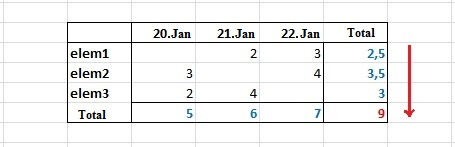Later, I found a “more correct” solution. I created Named Set.

I rewrote Calculated Member as follows:

Thus, a copy of the [ELEM COPY] measurement was no longer needed.

I presented this solution to the customer and got busy with other tasks. After some time, I received a new technical assignment, which featured a “statistical average”. To my question – What is this? He said that users do not need the sum of the average values for each element for the period, but the period average, that is, a usual Average of Children, but he asked me to keep my solution.

Additionally, he asked to add the period average taking into consideration all the days, not just those in which there are values.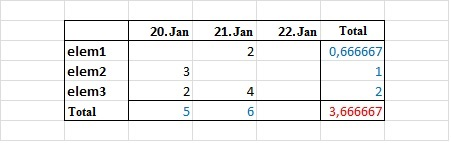(5 + 6) / 3 = 3.666667

I solved the task with the help of an additional fact, which simply counted the number of lines in the table for Time Dimension (in this solution, there is always one record for each day in this table). Further, I added the facts with aggregation – sum (AggregateFunction = Sum) and Calculated Member, in which the Sum was divided by the number of days.

Storage

The client we work for is not so simple-minded. After we had dealt with the Cube, we faced a more global task – to develop a storage (Data Vault), but not a simple one. The first thing we were told is that now our Bible is “Building a Scalable Data Warehouse with Data Vault 2.0” written by Daniel Linstedt and insisted on adding “Bitemporal Database Table Design“. We were to build a cube with historicity based on the constructed storage. This cube has two time dimensions, one shows a business date, the other – a transaction date. If we talk about the development process, it was painful, even now it hurts, but it worked.

The essence of “Bitemporal Database Table Design” is that each record has 4 additional fields:

3. Transaction_date_from
4. Transaction_date_to

The first two contain the interval of business dates – from which date and by which date a value did not change. The other 2 fields contain the interval of transaction dates. The period during which the value was not adjusted (as an option ante-dated) is stored in this interval.

One of the problems in solving this task was the source data, more precisely the form in which we received them. Data came in the form of daily slices. That is, there was the Date field which stored the date, the next day, a new value was in the Date field and the data either changed that day or not. It was required to combine the data into periods. If, for example, a value remained unchanged for 3 days in a row, 3 lines must be saved as one that would have two columns BEGIN and END, instead of column DATA.

Data before conversion: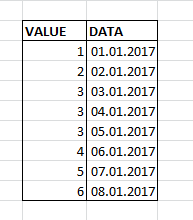Data after conversion: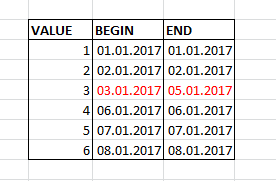I solved this problem using functions LAG and LEAD. The essence of the solution is that if a value is not changed for 3 days in a row, the date from the current line minus the date from the previous one will equal 1: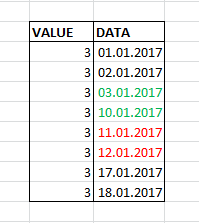• If the data are in succession, then 01.2017 – 11.01.2017 = 1
• If there is a gap between the data, then 01.2017 – 03.01.2017 = 7

The result is:

Next, we must somehow group this result, combine the pairs for those periods that lasted more than one day and leave those, the period of which lasted one day.

I did the grouping, numbered the lines and combined the even and odd ones.

Thus, the whole query is:

Summary:

In this article, I told only about the most, in my opinion, interesting things that were in the project. In addition, there was a lot of routine, disputes and other, not so original, solutions. I hope that the things I wrote about will be interesting and useful.